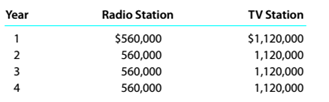Chapter 15, Problem 15.4.3P

Chapter
Section
Textbook Problem

Net present value method, internal rate of return method, and analysis The management of 1-leckel Communications Inc. is considering two capital investmentprojects. The estimated net cash flows from each project are as follows:The radio station requires an investment of $1,598800, while the TV station requiresan investment of$3,401,440. No residual value is expected from either project. InstructionsWhat advantage does the internal rate of return method have over the net present valuemethod ¡n comparing projects?

To determine

Concept Introduction:

IRR:

Internal Rate of Return (IRR) is the rate at which the NPV of the project is 0 or we can say that IRR is the rate of return at which the project is at breakeven. IRR is calculated using excel or approximation method.

NPV:

Net present value (NPV) is the method to evaluate the project feasibility. This method calculates the present value of cash inflows and outflows, and then calculates the net present value of the investment. A project should be accepted if it has a positive NPV. The formula to calculate the NPV is as follows:

NPV = Present value of cash inflows  Present value of cash out flows

To Indicate:

The benefit of Internal Rate of return method over the Net present value method for the evaluation of capital investment proposals

Explanation

Internal Rate of Return (IRR) is the rate at which the NPV of the project is 0 or we can say that IRR is the rate of return at which the project is at breakeven. IRR is calculated using excel or approximation method.

Net present value (NPV) is the method to evaluate the project feasibility...

Still sussing out bartleby?

Check out a sample textbook solution.

See a sample solution

The Solution to Your Study Problems

Bartleby provides explanations to thousands of textbook problems written by our experts, many with advanced degrees!

Get Started

Find more solutions based on key concepts